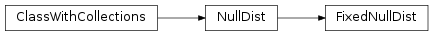# mvpa2.clfs.stats.FixedNullDist¶class `mvpa2.clfs.stats.``FixedNullDist`(dist, **kwargs)

All distributions from SciPy’s ‘stats’ module can be used with this class.

Examples

```>>> import numpy as np
>>> from scipy import stats
>>> from mvpa2.clfs.stats import FixedNullDist
>>>
>>> dist = FixedNullDist(stats.norm(loc=2, scale=4), tail='left')
>>> dist.p(2)
0.5
>>>
>>> dist.cdf(np.arange(5))
array([ 0.30853754,  0.40129367,  0.5       ,  0.59870633,  0.69146246])
>>>
>>> dist = FixedNullDist(stats.norm(loc=2, scale=4), tail='right')
>>> dist.p(np.arange(5))
array([ 0.69146246,  0.59870633,  0.5       ,  0.40129367,  0.30853754])
```

Attributes

 `descr` Description of the object if any `tail`

Methods

 `cdf`(x) Return value of the cumulative distribution function at `x`. `dists`() Implementations returns a sequence of the `dist_class` instances that were used to fit the distribution. `fit`(measure, ds) Does nothing since the distribution is already fixed. `p`(x[, return_tails]) Returns the p-value for values of `x`. `rcdf`(x) Implementations return the value of the reverse cumulative distribution function. `reset`()
Parameters: dist : distribution object This can be any object the has a `cdf()` method to report the cumulative distribition function values. enable_ca : None or list of str Names of the conditional attributes which should be enabled in addition to the default ones disable_ca : None or list of str Names of the conditional attributes which should be disabled tail : {‘left’, ‘right’, ‘any’, ‘both’} Which tail of the distribution to report. For ‘any’ and ‘both’ it chooses the tail it belongs to based on the comparison to p=0.5. In the case of ‘any’ significance is taken like in a one-tailed test. descr : str Description of the instance

Attributes

 `descr` Description of the object if any `tail`

Methods

 `cdf`(x) Return value of the cumulative distribution function at `x`. `dists`() Implementations returns a sequence of the `dist_class` instances that were used to fit the distribution. `fit`(measure, ds) Does nothing since the distribution is already fixed. `p`(x[, return_tails]) Returns the p-value for values of `x`. `rcdf`(x) Implementations return the value of the reverse cumulative distribution function. `reset`()
`cdf`(x)

Return value of the cumulative distribution function at `x`.

`fit`(measure, ds)

Does nothing since the distribution is already fixed.